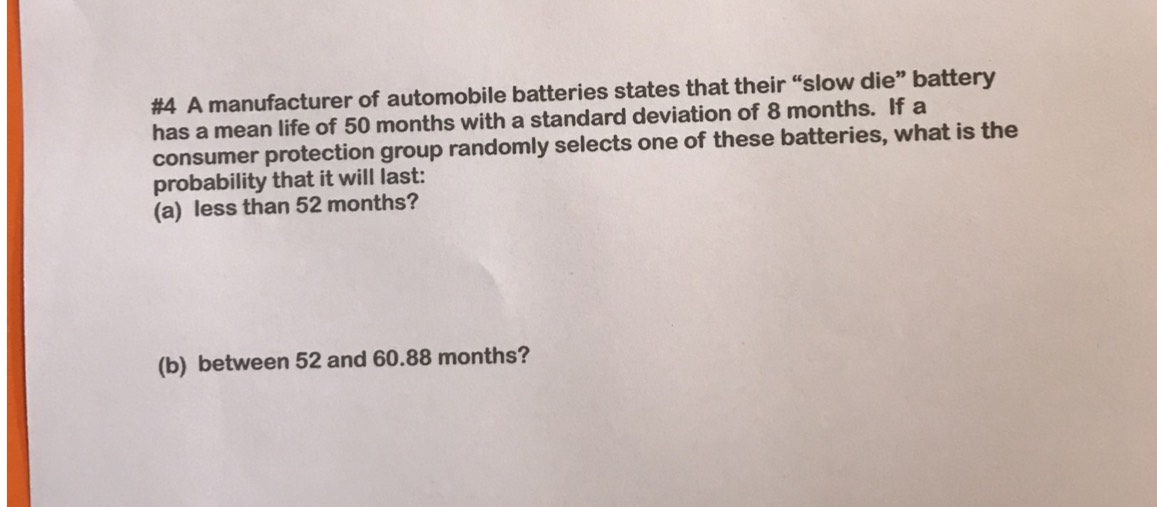### ¿Todavía tienes preguntas de matemáticas?

Pregunte a nuestros tutores expertos
Algebra
Pregunta#4 A manufacturer of automobile batteries states that their "slow die" battery has a mean life of $$50$$ months with a standard deviation of $$8$$ months. If a consumer protection group randomly selects one of these batteries, what is the probability that it will last:

(a) less than $$52$$ months?

(b) between $$52$$ and $$60.88$$ months?